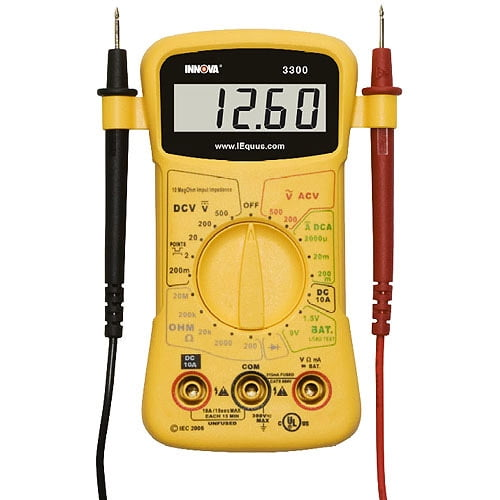# Motor current calculation using Winding resistance

### Motor current calculation:

The relationship between current (measured in amperes), resistance (measured in ohms) and voltage is found in one of the basic laws of electricity and electronics: Ohm’s Law. Ohm’s Law says that current is directly proportional to voltage and inversely proportional to resistance. This means that, if we know the resistance of a circuit, like the windings of a motor, and we know the voltage being applied to the circuit, we can calculate the current of the motor.

V= IR,

V-voltage, I- current , R- resistanceFind out the voltage entering the windings of the motor, either by using the volt-ohm meter to determine how many volts are being supplied to the motor or by reading the voltage of the power supply line from specifications, tags, warnings or other sources.

Find out the resistance of the motor’s windings. You can test this with the volt-ohm meter at the motor’s brushes, with the motor switched off and isolated from the power supply by an open switch or otherwise disconnected from the power supply.Divide the number of volts by the number of ohms of resistance; the answer is the amount of current, measured in amperes, or amps.

For example, if the power supply is providing 120 volts to the motor and the resistance of the windings is 12 ohms, divide 120 by 12 to get 10 amps, the amount of current the motor is using.